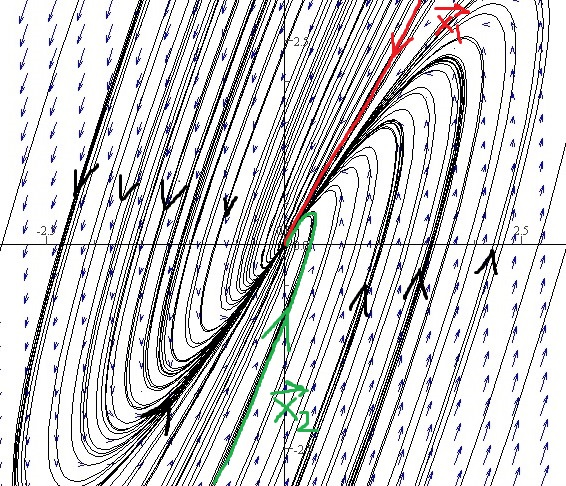DiffEq Home

## Repeated Eigenvalues for Linear Systems

Consider the following linear system: $\overrightarrow{x}'=A\overrightarrow{x}, \;\;\;\;(56)$ where $$A$$ is a $$2\times 2$$ real matrix. If $$A$$ has two distinct eigenvalues, then there are two linearly independent eigenvectors giving two linearly independent solution of (56). But if $$A$$ has only one distinct eigenvalue $$\lambda$$ of multiplicity 2, then there is only one linearly independent eigenvector $$\overrightarrow{v}$$ giving only one linearly independent solution $$\overrightarrow{x}=e^{\lambda t}\overrightarrow{v}$$ of (56). It can be verified that $$\overrightarrow{x}=te^{\lambda t}\overrightarrow{v}$$ is not a nonzero solution of (56).

Theorem. Suppose $$\lambda$$ is an eigenvalue of a $$2\times 2$$ real matrix $$A$$ of multiplicity 2 with an eigenvector $$\overrightarrow{v}$$. Then the general solution of $$\overrightarrow{x}'=A\overrightarrow{x}$$ is $\overrightarrow{x}=c_1e^{\lambda t}\overrightarrow{v}+c_2(te^{\lambda t}\overrightarrow{v}+e^{\lambda t}\overrightarrow{w}),$ where $$(A-\lambda I)\overrightarrow{w}=\overrightarrow{v}$$ or, equivalently $$(A-\lambda I)^2\overrightarrow{w}=\overrightarrow{0}$$. ($$\overrightarrow{w}$$ is called a generalized eigenvector of $$A$$ corresponding the eigenvalue $$\lambda$$)

First we show that $$\overrightarrow{x}=te^{\lambda t}\overrightarrow{v}+e^{\lambda t}\overrightarrow{w}$$ is a solution of $$\overrightarrow{x}'=A\overrightarrow{x}$$. \begin{align*} LHS&=(te^{\lambda t}\overrightarrow{v}+e^{\lambda t}\overrightarrow{w})'=e^{\lambda t}\overrightarrow{v}+t\lambda e^{\lambda t}\overrightarrow{v}+\lambda e^{\lambda t}\overrightarrow{w}\\ RHS&=A(te^{\lambda t}\overrightarrow{v}+e^{\lambda t}\overrightarrow{w})=te^{\lambda t}A\overrightarrow{v}+e^{\lambda t}A\overrightarrow{w} =te^{\lambda t}\lambda\overrightarrow{v}+e^{\lambda t}(\lambda\overrightarrow{w}+\overrightarrow{v}), \end{align*} since $$(A-\lambda I)\overrightarrow{w}=\overrightarrow{v}$$, i.e., $$A\overrightarrow{w}=\lambda\overrightarrow{w}+\overrightarrow{v}$$. Thus $$LHS=RHS$$. Using Wronskian it can be shown that these two solutions are linearly independent.

Example. $$\overrightarrow{x}'=\left[\begin{array}{rr} 1&-1\\4&-3\end{array} \right] \overrightarrow{x}$$.

1. Find the general solution.

2. Draw the phase portrait.

Solution. (a) The eigenvalues of the coefficient matrix are $$-1$$ and $$-1$$ with corresponding eigenvector $$\overrightarrow{v}=\left[\begin{array}{r}1\\2\end{array} \right]$$ (show all the steps). Now let's find a vector $$\overrightarrow{w}$$ that satisfies \begin{align*} &(A-\lambda I)\overrightarrow{w}=\overrightarrow{v}\\ \implies & \left[\begin{array}{rr} 2&-1\\4&-2\end{array} \right] \left[\begin{array}{r}w_1\\w_2\end{array} \right] = \left[\begin{array}{r}1\\2\end{array} \right]\\ \implies & \left[\begin{array}{r}2w_1-w_2\\4w_1-2w_2\end{array} \right] = \left[\begin{array}{r}1\\2\end{array} \right]\\ \implies & 2w_1-w_2=1\\ \implies & w_2=2w_1-1\\ \implies & \overrightarrow{w}=\left[\begin{array}{r}w_1\\w_2\end{array} \right] = \left[\begin{array}{l}w_1\\2w_1-1\end{array} \right] = w_1\left[\begin{array}{r}1\\2\end{array} \right] +\left[\begin{array}{r}0\\-1\end{array} \right] \end{align*} Assigning a particular value of $$w_1$$, say $$w_1=0$$, we get $$\overrightarrow{w}=\left[\begin{array}{r}0\\-1\end{array} \right]$$. So the general solution is $\overrightarrow{x}=c_1e^{-t}\left[\begin{array}{r}1\\2\end{array} \right]+c_2\left(te^{-t}\left[\begin{array}{r}1\\2\end{array} \right]+e^{-t}\left[\begin{array}{r}0\\-1\end{array} \right]\right).$ (b) To draw the curve for $$\overrightarrow{x_2}$$, get three points on it by plugging $$t=-1,0,1$$. The origin is called an improper node which is asymptotically stable when eigenvalues are negative (trajectories move toward the origin as $$t$$ increases) and unstable when eigenvalues are positive (trajectories move away from the origin as $$t$$ increases).Note:
If $$\overrightarrow{x}'=A \overrightarrow{x}$$ has a solution $$\overrightarrow{x}_2=te^{-t}\left[\begin{array}{r}1\\2\end{array} \right]+e^{-t}\left[\begin{array}{r}0\\-1\end{array} \right]$$ where $$A$$ is not given. Since $$\overrightarrow{x}_2(0)=\left[\begin{array}{r}0\\-1\end{array} \right]$$, the solution curve passes through $$(0,-1)$$. To find its direction at $$(0,-1)$$, find the tangent vector $$\overrightarrow{x}'_2 =(1\cdot e^{-t}-te^{-t})\left[\begin{array}{r}1\\2\end{array} \right]-e^{-t}\left[\begin{array}{r}0\\-1\end{array} \right] =\left[\begin{array}{r}1\\3\end{array} \right]$$ at $$t=0$$.

Last edited